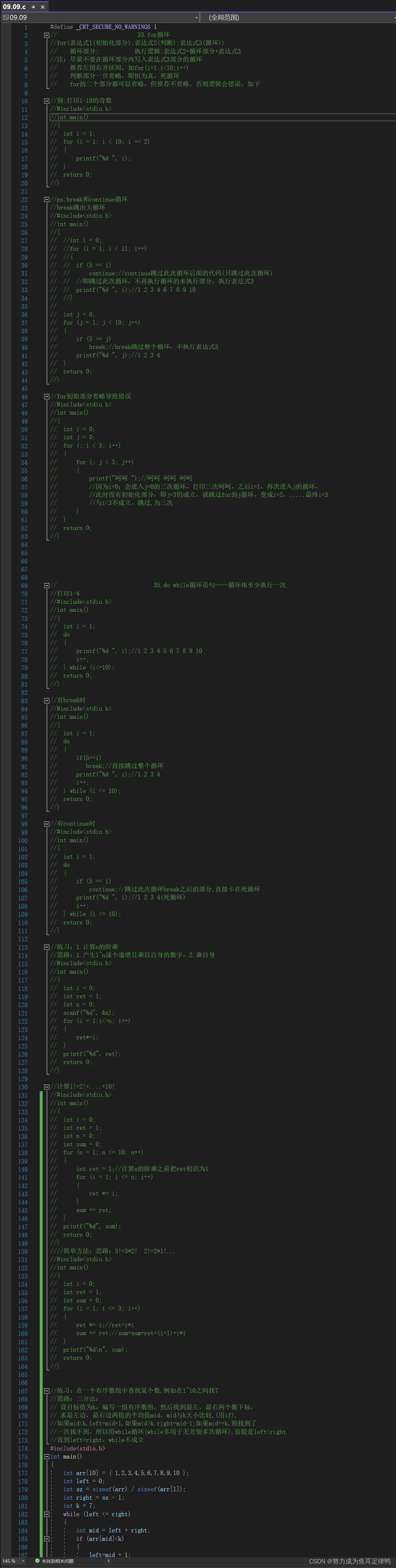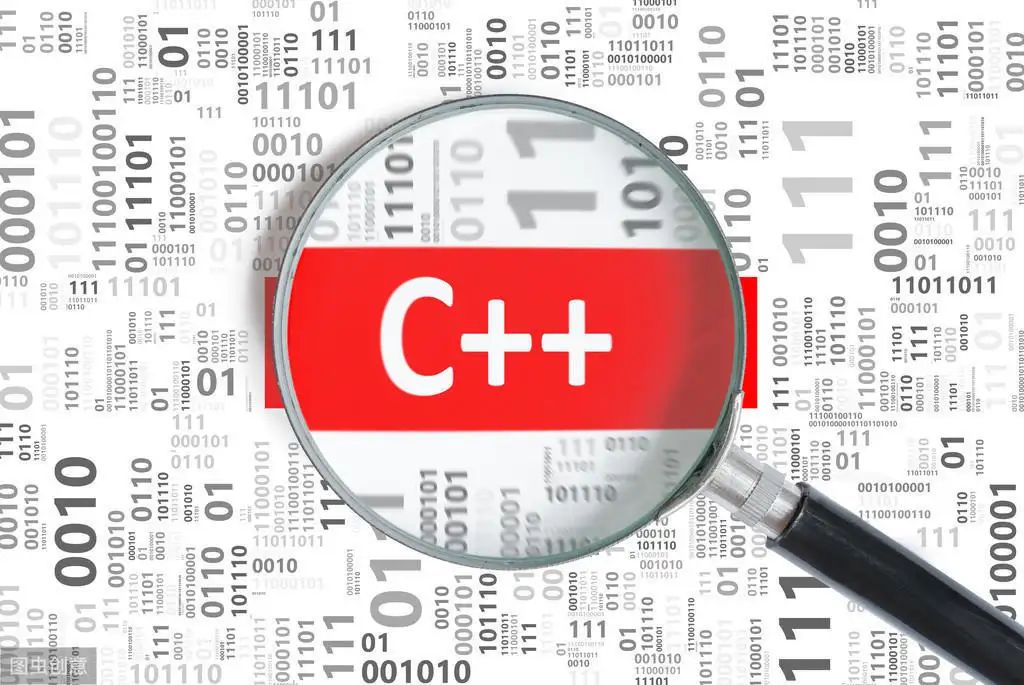# 斐波那契数列和反向计算问题

+关注继续查看

 1 #include <stdio.h>
2 #include <stdlib.h>
3 void toBinary(unsigned long);
4 int main()
5 {
6     unsigned long num;
7     printf("输入一个正整数：q退出\n");
8     while(scanf_s("%ul", &num) == 1)
9     {
10         toBinary(num);
11         putchar('\n');
12         printf("输入一个正整数：\n");
13     }
14     system("pause");
15     return 0;
16 }
17 //递归函数
18 void toBinary(unsigned long num)
19 {
20     int r;
21     r = num % 2;//最后一位要输出的，即使参数=1，还是要计算到这里结束，只取出余数就ok了。然后顺次返回上一级主调函数，继续执行剩下的……
22     //如果商 1 / 2 = 0，计算就可以终止了，不需要再算
23     if (num >= 2)
24     {
25         //精华，联系10进制转2进制的算法，每次除以2，取出余数，然后用新的商继续除以2，取出新余数……直到商为0，余数逆序输出即可
26         toBinary(num / 2);//把新的商作为参数递归调用
27     }
28     //在递归语句之后输出，这样就是倒叙输出
29     printf("%d", r);
30 }

//将无符号十进制数转化为标准16位二进制数
int main()
{
unsigned short i;

cout << "请输入一个小于65536的正整数" << endl;
cin >> i;
//for语句从15递减到0，一共16次对二进制数的每一位的判断作操作。
for(int j = 15; j >= 0; j--)
{
//<<运算表示把1的二进制形式整体向左移j位，左移后低位补0，移出的高位部分被舍弃。
if (i & (1 << j)){
//i&（1<<j）的值相当于把i的二进制的第j位取出来（i的第j位与（1<<j）的第j位与，i的第j位为1时值为真）循环后既得i的二进制形式。
cout << "1";
}
else{
cout << "0";
}
}

cout << endl;

return 0;
}

<<运算表示把1的二进制形式整体向左移j位，左移后低位补0，移出的高位部分被舍弃。

_itoa(120, &buffer, 16); 第一个参数是要转换的数，第二个是地址，第三个是进制数

F1=F2=1

long long fibonacciRecursion(unsigned int n ){
if (0 == n){
return 0;
}
else if (1 == n){
return 1;
}
else{
return fibonacciRecursion(n - 1) + fibonacciRecursion(n - 2);
}
}//递推的解法，非递归
long long fibonacciRecursion(unsigned int n ){
//先判断 n=0和1的时候
if (0 == n){
return 0;
}
else if (1 == n){
return 1;
}
else
{
long n0 = 0;
long n1 = 1;
long fn = 0;
for (int i = 2; i <= n; i++){
fn = n1 + n0;
n0 = n1;
n1 = fn;//这是递推的过程
}

return fn;
}
}http://www.cnblogs.com/kubixuesheng/p/4385682.html12 042 0[解题报告]【第03题】给定 a 和 b ，交换它们的值并输出 | 四种解法
[解题报告]【第03题】给定 a 和 b ，交换它们的值并输出 | 四种解法
71 07种方式实现斐波那契数列
1257 01363 01082 0713 0【递归】斐波那契数列第n个数

978 0